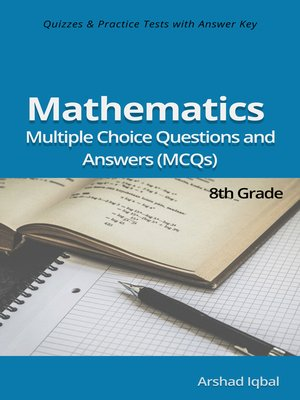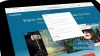### Multiple Choice Questions and Answers (Quiz & Tests with Answer Keys)

ebook

With an OverDrive account, you can save your favorite libraries for at-a-glance information about availability. Find out more about OverDrive accounts.

### Subjects

8th grade math multiple choice questions has 330 MCQs. Grade 8 math quiz questions and answers pdf, MCQs on math applications, trigonometry and trigonometry formulas, trigonometric ratios, coordinate geometry, basic mathematics MCQs with answers, indices and standard form, linear inequalities, compound inequalities, mensuration formulas, arc length and sector area, radian measure MCQs and quiz to practice exam prep tests.
8th grade math multiple choice quiz questions and answers pdf, math exam revision and study guide with practice tests for online exam prep and interviews. Math interview questions and answers to ask, to prepare and to study for jobs interviews and career MCQs with answer keys.
Coordinate geometry quiz has 15 multiple choice questions. Indices and standard form quiz has 116 multiple choice questions. Linear inequalities quiz has 26 multiple choice questions. Math applications quiz has 49 multiple choice questions with answers. Mensuration arc length, sector area and radian measure quiz has 31 multiple choice questions. Trigonometric ratios quiz has 56 multiple choice questions. Trigonometry and trigonometry formulas quiz has 37 multiple choice questions.
Math interview questions and answers pdf, MCQs on angles and circle, angles and trigonometrical ratio, applications of trigonometry, arc length and area of sector, area of triangle, circle area and circumference, common prefixes, compound interest, cosine rule, division law of indices, everyday math, fractional indices, hire purchase, indices laws, inequalities, length of line segment, math applications, math prefixes, math symbols, money exchange, multiplication law of indices, percentage calculations, personal and household finances, power law of indices, practical trigonometry applications, problem solving inequalities, profit and loss percentage, radian (rad), radian to degree conversion, sine rule and formula, solving inequalities, solving right angled triangles, symmetrical properties of circles, taxation, three dimensional problems, trigonometrical ratios, use of simple calculator, zero and negative indices, grade 8 math worksheets for competitive exams preparation.

### Publication Details

Publisher:
Imprint:
Smashwords Edition
Publication Date:
2016

### Format